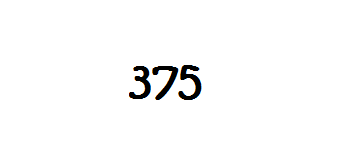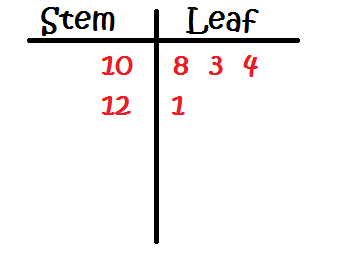# 3-Digit Stem-and-Leaf Plots

Instructions:

question 1 of 3

### What does a stem-and-leaf plot contain?

Create Your Account To Take This Quiz

As a member, you'll also get unlimited access to over 79,000 lessons in math, English, science, history, and more. Plus, get practice tests, quizzes, and personalized coaching to help you succeed.

Try it risk-free for 30 days. Cancel anytime

### 1. In the following number, which digit(s) are the 'stem' and which are the 'leaf?'### 2. Mark created a stem-and-leaf plot to show his last four video game scores. What were his scores?Create your account to access this entire worksheet
Quizzes, practice exams & worksheets
Certificate of Completion
Create an account to get started

For this quiz and worksheet combination, you will be looking at three-digit-long stem-and-leaf plots. Questions focus on how to properly draw a stem-and-leaf plot and numbers used to create an example plot.

## Quiz & Worksheet Goals

You can find these topics inside the questions of the quiz:

• Identifying the stem and the leaf
• The number of stems with given numbers
• How to properly draw a plot
• Using specific numbers to create a plot

## Skills Practiced

• Knowledge application - use what you know to answer questions about the drawing of a stem-and-leaf plot
• Information recall - remember what you have learned about the digits that make up the stem and leaf
• Making connections - see how a given set of numbers are turned into a plot

For more practice with plots, open the lesson titled 3-Digit Stem-and-Leaf Plots. With it, you are able to check out the extra content which includes the following:

• Creating a plot